# Star

Calculate the content of the shaded part of square with side 28 cm.

S =  168.2478 cm2

### Step-by-step explanation: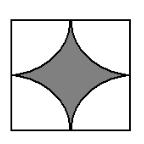Did you find an error or inaccuracy? Feel free to write us. Thank you!#### You need to know the following knowledge to solve this word math problem:

We encourage you to watch this tutorial video on this math problem:

## Related math problems and questions:

• 6 regular polygonIt is given 6 side regular polygon whose side is 5 cm. Calculate its content area. Compare how many more cm2 (square centimeters) has a circle in which is inscribed the 6-gon.
• Calculate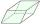Calculate the content cm2 diamond with side length 9.8 cm and a height on the side of 5 cm.
• Annulus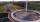Calculate the area of two circles annulus k1 (S, 3 cm) and k2 (S, 5 cm).
• Tripled squareIf you tripled the length of the sides of the square ABCD you increases its content by 200 cm2. How long is the side of the square ABCD?
• 22/7 circleCalculate approximately the area of a circle with radius 20 cm. When calculating π, use 22/7.
• Annulus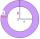Two concentric circles form an annulus of width 10 cm. The radius of the smaller circle is 20 cm. Calculate the content area of the annulus.
• Math heart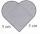Stylized heart shape created from a square with side 5 cm and two semicircles over his sides. Calculate the content area and its circumference.
• Find the 28Find the area of a square with a side length of 4/5 yards.
• Square and circle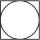Into square is an inscribed circle with diameter 10 cm. What is the difference between circumference square and circle?
• Tiler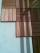On what surface m2 tiler must lay 30 square tiles, each of which has a side 25 cm?
• A butterA butter cube with an edge 6.5 cm long is packed in a package with dimensions a = 28 cm, b = 15 cm. Calculate how many cm2 the package is larger than the surface of the cube.
• Triangle eqCalculate accurate to hundredths cm height of an equilateral triangle with a side length 12 cm. Calculate also its perimeter and content area.Calculate the radius of the quadrant, which area is equal to area of circle with radius r = 15 cm.Calculate the content of a shape in which an arbitrary point is not more than 3 cm from the segment AB. The length of the segment AB is 5 cm.Gift box has a rectangular shape with dimensions of 8×8×3 cm. Miloslav wants to cover with square paper with sides of 18 cm. How much paper left him?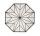Octagonal mat formed from a square plate with a side of 40 cm so that every corner cut the isosceles triangle with leg 3.6 cm. What is the content area of one mat?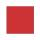The side length of the square is 54 cm. How many times increases the content area of a square if the length of side increase three times?